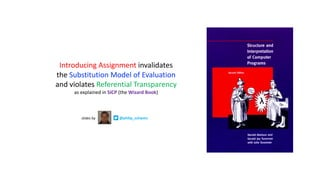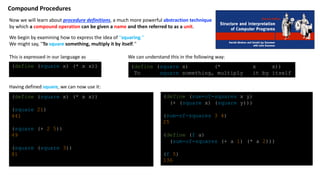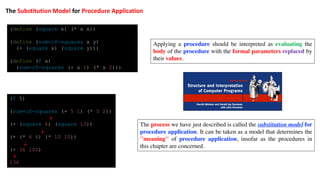Se está descargando tu SlideShare. ×

# Introducing Assignment invalidates the Substitution Model of Evaluation and violates Referential Transparency - as explained in SICP (the Wizard Book)

Anuncio
Anuncio
Anuncio
Anuncio
Anuncio
Anuncio
Anuncio
Anuncio
Anuncio
Anuncio
AnuncioCargando en…3
×

1 de 6 Anuncio

# Introducing Assignment invalidates the Substitution Model of Evaluation and violates Referential Transparency - as explained in SICP (the Wizard Book)

Introducing Assignment invalidates the Substitution Model of Evaluation and violates Referential Transparency
- as explained in SICP (the Wizard Book)

Introducing Assignment invalidates the Substitution Model of Evaluation and violates Referential Transparency
- as explained in SICP (the Wizard Book)

Anuncio
Anuncio

### Introducing Assignment invalidates the Substitution Model of Evaluation and violates Referential Transparency - as explained in SICP (the Wizard Book)

1. 1. Introducing Assignment invalidates the Substitution Model of Evaluation and violates Referential Transparency as explained in SICP (the Wizard Book) @philip_schwarzslides by
2. 2. (define (square x) (* x x)) To square something, multiply it by itself (define (square x) (* x x)) (square 21) 441 (square (+ 2 5)) 49 (square (square 3)) 81 Now we will learn about procedure definitions, a much more powerful abstraction technique by which a compound operation can be given a name and then referred to as a unit. (define (square x) (* x x)) We can understand this in the following way: We begin by examining how to express the idea of ''squaring.'' We might say, ''To square something, multiply it by itself.'' Compound Procedures Having defined square, we can now use it: (define (sum-of-squares x y) (+ (square x) (square y))) (sum-of-squares 3 4) 25 (define (f a) (sum-of-squares (+ a 1) (* a 2))) (f 5) 136 This is expressed in our language as
3. 3. (f 5) (sum-of-squares (+ 5 1) (* 5 2)) (+ (square 6) (square 10)) (+ (* 6 6) (* 10 10)) (+ 36 100) 136 (define (square x) (* x x)) (define (sum-of-squares x y) (+ (square x) (square y))) (define (f a) (sum-of-squares (+ a 1) (* a 2))) The process we have just described is called the substitution model for procedure application. It can be taken as a model that determines the ''meaning'' of procedure application, insofar as the procedures in this chapter are concerned. Applying a procedure should be interpreted as evaluating the body of the procedure with the formal parameters replaced by their values. The Substitution Model for Procedure Application
4. 4. (define acc (make-account 100)) ((acc 'withdraw) 25) 75 ((acc 'withdraw) 25) 50 ((acc 'withdraw) 60) "Insufficient funds" ((acc 'deposit) 40) 90 ((acc 'withdraw) 60) 30 Local State Variables To illustrate what we mean by having a computational object with time-varying state, let us model a bank account. We should obtain the following sequence of responses: (define (make-account balance) (define (withdraw amount) (if (>= balance amount) (begin (set! balance (- balance amount)) balance) "Insufficient funds")) (define (deposit amount) (set! balance (+ balance amount)) balance) (define (dispatch m) (cond ((eq? m 'withdraw) withdraw) ((eq? m 'deposit) deposit) (else (error "Unknown request -- MAKE-ACCOUNT" m)))) dispatch) Combining set! with local variables is the general programming technique we will use for constructing computational objects with local state. Unfortunately, using this technique raises a serious problem: When we first introduced procedures, we also introduced the substitution model of evaluation to provide an interpretation of what procedure application means. We said that applying a procedure should be interpreted as evaluating the body of the procedure with the formal parameters replaced by their values. The trouble is that, as soon as we introduce assignment into our language, substitution is no longer an adequate model of procedure application. This uses the set! special form, whose syntax is (set! <name> <new-value>) Here <name> is a symbol and <new-value> is any expression. set! changes <name> so that its value is the result obtained by evaluating <new-value>. In the case at hand, we are changing balance so that its new value will be the result of subtracting amount from the previous value of balance. Observe that the expression ((acc 'withdraw) 25) evaluated twice, yields different values. This is a new kind of behavior for a procedure. Until now, all our procedures could be viewed as specifications for computing mathematical functions. A call to a procedure computed the value of the function applied to the given arguments, and two calls to the same procedure with the same arguments always produced the same result. Actually, this is not quite true. One exception was the random-number generator in section ... Another exception involved ... On the other hand, until we introduce assignment, we have no way to create such procedures ourselves. Here is a procedure that returns a ''bank-account object'' with a specified initial balance: introducing assignment
5. 5. As we have seen, the set! operation enables us to model objects that have local state. However, this advantage comes at a price. Our programming language can no longer be interpreted in terms of the substitution model of procedure application that we introduced in section 1.1.5. Moreover, no simple model with ''nice'' mathematical properties can be an adequate framework for dealing with objects and assignment in programming languages. So long as we do not use assignments, two evaluations of the same procedure with the same arguments will produce the same result, so that procedures can be viewed as computing mathematical functions. Programming without any use of assignments, as we did throughout the first two chapters of this book, is accordingly known as functional programming. (define (create-account-decrementer! balance) (lambda (amount) (set! balance (- balance amount)) balance)) ((create-account-decrementer 25) 20) ((lambda (amount) (- 25 amount)) 20) (- 25 20) 5 If we adhered to the substitution model, we would have to say that the meaning of the procedure application is to first set! balance to 5 and then return 25 as the value of the expression. This gets the wrong answer. In order to get the correct answer, we would have to somehow distinguish the first occurrence of balance (before the effect of the set!) from the second occurrence of balance (after the effect of the set!), and the substitution model cannot do this. The trouble here is that substitution is based ultimately on the notion that the symbols in our language are essentially names for values. But as soon as we introduce set! and the idea that the value of a variable can change, a variable can no longer be simply a name. Now a variable somehow refers to a place where a value can be stored, and the value stored at this place can change. we can use the substitution model to explain how create-account-decrementer works (define (create-account-decrementer balance) (lambda (amount) (- balance amount))) (define account-decrementer (create-account-decrementer 25)) (account-decrementer 20) 5 (account-decrementer 10) 15 (define account-decrementer! (create-account-decrementer! 25)) (account-decrementer! 20) 5 (account-decrementer! 10) - 5 ((create-account-decrementer! 25) 20) ((lambda (amount) (set! balance (- 25 amount)) 25) 20) (set! balance (- 25 20)) 25 25 The Cost of Introducing Assignment if we attempt a similar substitution analysis with create- account-decrementer!, we get the wrong answer. Does not use set! Uses set! programming without assignments is Functional Programming
6. 6. The issue surfacing here is more profound than the mere breakdown of a particular model of computation. As soon as we introduce change into our computational models, many notions that were previously straightforward become problematical. Consider the concept of two things being the "same." Suppose we call create-account-decrementer twice with the same argument to create two procedures: (define account-decrementer-1 (create-account-decrementer 25)) (define account-decrementer-2 (create-account-decrementer 25)) In fact, account-decrementer-1 could be substituted for account-decrementer-2 in any computation without changing the result. (define account-decrementer-1!(create-account-decrementer! 25)) (define account-decrementer-2! create-account-decrementer! 25)) (account-decrementer-1! 20) 5 (account-decrementer-1! 20) - 15 (account-decrementer-2! 20) 5 Even though account-decrementer-1! and account-decrementer-2! are ''equal'' in the sense that they are both created by evaluating the same expression, (create-account-decrementer! 25), it is not true that account-decrementer-1! could be substituted for account-decrementer-2! in any expression without changing the result of evaluating the expression. A language that supports the concept that ''equals can be substituted for equals'' in an expression without changing the value of the expression is said to be referentially transparent. Referential transparency is violated when we include set!in our computer language. This makes it tricky to determine when we can simplify expressions by substituting equivalent expressions. Consequently, reasoning about programs that use assignment becomes drastically more difficult. Are account-decrementer-1 and account-decrementer-2 the same? Contrast this with making two calls to create-account-decrementer!: Are account-decrementer-1! and account-decrementer-2! the same? Surely not, because calls to account-decrementer-1! and account-decrementer-2! have distinct effects, as shown by the following sequence of interactions: An acceptable answer is yes, because account-decrementer-1 and account-decrementer-2 have the same computational behavior -- each is a procedure that subtracts its input from 25. Sameness and Change introducing assignment violates Referential Transparency